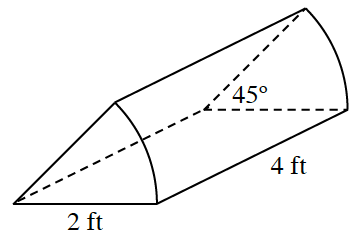### Home > CCG > Chapter 10 > Lesson 10.1.3 > Problem10-35

10-35.Compute the volume of the solid shown at right.

Think of the problem as trying to find $\frac{45}{360}$ of the volume of the cylinder.

Find the area of the sector.

$\pi\left(2\ \text{ft}\right)^2\left(\frac{45°}{360°}\right)=\frac{180\pi}{360}\text{ft}^2=\frac{\pi}{2}\text{ft}^2$

Multiply the area of the sector by the length of the solid.

$\frac{\pi}{2}\text{ft}^2\left(4\ \text{ft}\right)=\frac{4\pi}{2}\text{ft}^3=2\pi\ \text{ft}^3$

$≈ 6.28\text{ ft}^³$